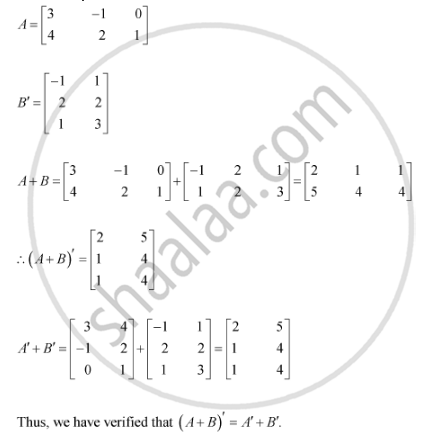Share

# If A' Matric [(3,4),(-1, 2),(0,1)] and B =Matric [((-1,2,1),(1,2,3))] Then Verify that (A + B)' = A' + B' - CBSE (Science) Class 12 - Mathematics

ConceptSymmetric and Skew Symmetric Matrices

#### Question

if A' [(3,4),(-1, 2),(0,1)] and B = [((-1,2,1),(1,2,3))] then verify that (A + B)' = A' + B'

#### Solution

It is known that A = (A')'

Therefore, we have:Is there an error in this question or solution?

#### Video TutorialsVIEW ALL 

Solution If A' Matric [(3,4),(-1, 2),(0,1)] and B =Matric [((-1,2,1),(1,2,3))] Then Verify that (A + B)' = A' + B' Concept: Symmetric and Skew Symmetric Matrices.
S